# 4.4.2: Vegetable Oil Hydrogenation, Trans Fats, and Percent Yield

$$\newcommand{\vecs}{\overset { \rightharpoonup} {\mathbf{#1}} }$$ $$\newcommand{\vecd}{\overset{-\!-\!\rightharpoonup}{\vphantom{a}\smash {#1}}}$$$$\newcommand{\id}{\mathrm{id}}$$ $$\newcommand{\Span}{\mathrm{span}}$$ $$\newcommand{\kernel}{\mathrm{null}\,}$$ $$\newcommand{\range}{\mathrm{range}\,}$$ $$\newcommand{\RealPart}{\mathrm{Re}}$$ $$\newcommand{\ImaginaryPart}{\mathrm{Im}}$$ $$\newcommand{\Argument}{\mathrm{Arg}}$$ $$\newcommand{\norm}{\| #1 \|}$$ $$\newcommand{\inner}{\langle #1, #2 \rangle}$$ $$\newcommand{\Span}{\mathrm{span}}$$ $$\newcommand{\id}{\mathrm{id}}$$ $$\newcommand{\Span}{\mathrm{span}}$$ $$\newcommand{\kernel}{\mathrm{null}\,}$$ $$\newcommand{\range}{\mathrm{range}\,}$$ $$\newcommand{\RealPart}{\mathrm{Re}}$$ $$\newcommand{\ImaginaryPart}{\mathrm{Im}}$$ $$\newcommand{\Argument}{\mathrm{Arg}}$$ $$\newcommand{\norm}{\| #1 \|}$$ $$\newcommand{\inner}{\langle #1, #2 \rangle}$$ $$\newcommand{\Span}{\mathrm{span}}$$$$\newcommand{\AA}{\unicode[.8,0]{x212B}}$$

Dietary intake of vegetable oils and hydrogenated vegetable oils has significant health effects. Not only do they have about twice as many calories per gram as sugars and proteins, but they have long term effects on circulatory system health. Crisco®, with saturated oils, may not be as healthy as olive oil, with more unsaturated oils.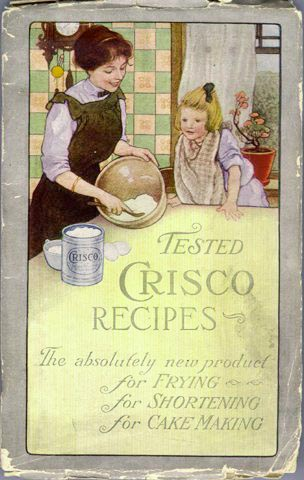Crisco contains hydrogenated vegetable oilolive oil is 55-83% oleic acid

To understand these effects, we need to look at the structure of triglycerides. The triglyceride  is an important part of the blood test done with an annual physical exam.

## Triglycerides

Vegetable oils are all triglycerides, which contain a glycerol (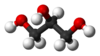) three carbon "backbone" with 3 long chain "fatty acids" attached through ester linkages, as in the figure below.Figure $$\PageIndex{1}$$: A triglyceride, overall unsaturated, with the glycerol "backbone" on the left, and saturated palmitic acid, monounsaturated oleic acid, and polyunsaturated alpha-linolenic acid.

The long chain fatty acids may be saturated with hydrogen atoms, in which case they have all single bonds like the top fatty acid in the Figure (which is palmitic acid). If they have fewer hydrogen atoms, they are unsaturated and have double bonds like the middle fatty acid in the Figure (which is oleic acid). The bottom fatty acid is polyunsaturated, with multiple double bonds (it is linolenic acid). Various cooking oils have |known concentrations of saturated and unsaturated fatty acids.

## Saturated vs. Unsaturated vs. Trans Fatty Acids

Generally, triglycerides with more unsaturated fatty acid substituents are more healthful, but food companies hydrogenate them to make them solid saturated fats (like margarine or Crisco), and to reduce the tendency to spoil. Unsaturated fats have kinks in their molecular structures that reduce the tendency for them to cause arthereosclerosis (clogged arteries), pretty much for the same reason that kinks reduce the tendency to pack efficiently and form solids. Saturated fats have more linear fatty acid chains that pack well and solidify easily. Compare the Jmol models of saturated palmitic acid and unsaturated oleic acid below. Partial hydrogenation of polyunsaturated fats also produces trans-fatty acids, which have structures like saturated fats and consequently are as unhealty (see Elaidic Acid Below):Figure $$\PageIndex{2}$$: Elaidic Acid, C18H34O2

Trans- fatty acids have the hydrogens on opposite sides of the C=C double bond, like thiswhile cis-fatty acids have the hydrogen atoms on the same side, like this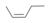.

## Composition of Crisco, "partially hydrogenated" vegetable oil

As of 2010 Crisco is made of soybean oil, fully hydrogenated cottonseed oil, and partially hydrogenated soybean and cottonseed oils. According to the product information label, one 12 g serving of Crisco contains 3 g of saturated fat, 0g of trans fat, 6 g of polyunsaturated fat, and 2.5 g of monounsaturated fat. Notice that the fat masses don't add up because the weights of glycerol are not included in the separately listed components. Trans fatty acids are now recognized as a major dietary risk factor for cardiovascular diseases, and the US FDA has revised food labeling requirements to include trans fats.

The composition of the soybean oil in Crisco is shown below.

Name Fatty Acid Structure Formula Percent
Palmitic AcidSkeletal structure of palmitic acid shows long unsaturated carbon chain with C O O H bond at the end. C16H32O2 10
Stearic AcidSkeletal structure of stearic acid shows long unsaturated carbon chain with C O O H bond at the end. C18H36O2 4
Oleic Acid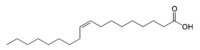Skeletal structure of oleic acid shows long unsaturated carbon chain with 1 double bond on the ninth carbon from the carbon of C O O H at the end. C18H34O2 23
Linoleic AcidSkeletal structure of linoleic acid shows long unsaturated carbon chain with 2 double bonds on the ninth and twelfth carbon from the carbon of C O O H at the end. C18H32O2 51
Linolenic Acid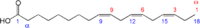Skeletal structure of linolenic acid shows long unsaturated carbon chain with 3 double bonds on the ninth, twelfth, and fifteenth carbon from the carbon of C O O H at the end. C18H30O2 7-10
Other     2-5

## Percent yield of hydrogenation products

Quite often a mixture of two or more products is formed in a chemical reaction. For example, when a vegetable oil like palm oil is hydrogenated, we might want to make just mono-unsaturated products. But the many triglycerides it contains with varied fatty acid chains. No single process could work for all of them. Suppose we start with just one possible palm oil molecule, a glycerol with 2 linolenic acid, and 1 linoleic acid substituents (we'll abbreviate it GLLL). The desired product might be the oil with three oleic acid substituents (we'll abbreviate it GOOO, which also might be a good description of it) so the equation is:

(C18H29O2)CH2CH(C18H29O2)CH2-(C18H31O2) + 5 H2 → (C18H33O2)CH2CH(C18H33O2)CH2-(C18H33O2)

"GLLL" + 5 H2 → "GOOO"

A large excess of hydrogen is usually present under pressure, with a palladium or "Raney Nickel" catalyst. A large number of products is obtained, including completely saturated fats like Stearin (glyceryl tristearate),and trans fats. The products are usually analyzed by converting the oils to simpler (methyl) esters and running a gas chromatogam.

The effectiveness of the reaction is usually evaluated in terms of percent yield of the desired product. A theoretical yield is calculated by assuming that all the limiting reagent is converted to product. The experimentally determined mass of product is then compared to the theoretical yield and expressed as a percentage:

$$\text{Percent yield}=\frac{\text{actual yield}}{\text{theoretical yield}}\times \text{100 percent}$$

##### Example $$\PageIndex{1}$$

Suppose a |hydrogenation of 100.0 g of (C18H29O2)CH2CH(C18H29O2)CH2-(C18H31O2), abbreviated "GLLL" (M = 875.4 g/mol) is carried out with 2.000 g H2, sealed in a high pressure steel reaction vessel with a catalyst at 55°C. The products include 90.96 g(C18H33O2)CH2CH(C18H33O2)CH2-(C18H33O2), abbreviated "GOOO" (M = 885.5 g/mol). Calculate the percent yield.

###### Solution

We must calculate the theoretical yield of (C18H33O2)CH2CH(C18H33O2)CH2-(C18H33O2), and to do this, we must first discover whether (C18H29O2)CH2CH(C18H29O2)CH2-(C18H31O2) or H2 is the limiting reagent. For the balanced equation above,

The stoichiometric ratio of the reactants is

$\text{S}\left( \frac{\text{GLLL}}{\text{H}_{\text{2}}} \right)=\frac{\text{1 mol GLLL}}{\text{5 mol H}_{\text{2}}} \nonumber$

Now, the initial amounts of the two reagents are and

\begin{align*} & n_{\text{GLLL}}\text{(initial)}=\text{100}\text{.0 g GLLL}\times \frac{\text{1 mol stearin}}{\text{875}\text{.4 g GLLL}}=\text{0}\text{.1142 mol GLLL} \\ & \\ & n_{\text{H}_2}\text{(initial)}=\text{2}\text{.000 g H}_2\times \frac{\text{1 mol H}_2}{\text{2}\text{.016 g H}_2}=\text{0}\text{.9921 mol H}_2 \\ \end{align*}

The ratio of initial amounts is thus

$\frac{n_{\text{GLLL}}\text{(initial)}}{n_{\text{H}_2}\text{(initial}} ~=~ \frac{\text{0}\text{.1142 mol stearin}}{\text{0}\text{.9921 mol H}_2} ~=~\frac{\text{0}\text{0.1151 mol stearin}}{\text{1 mol H}_2} \nonumber$

Since this ratio is less than $$\text{S}\left( \frac{\text{GLLL}}{\text{H}_2} \right)~=~0.20$$, there is an excess of H2. GLLL is the limiting reagent. Accordingly we must use 0.1142 mol GLLL and 0.5712 mol H2 (rather than 0.9921 mol H2) to calculate the theoretical yield of (C18H33O2)CH2CH(C18H33O2)CH2-(C18H33O2), or "GOOO". We then have

$n_{\text{GOOO}}\text{(theoretical)}=\text{0}\text{.1142 mol GLLL}\times \frac{\text{1 mol GOOO}}{\text{1 mol GLLL}}=\text{0}\text{.1142 mol GOOO} \nonumber$

so that

$\text{m}_{\text{GOOO}}\text{(theoretical)}=\text{0}\text{.1142 mol GOOO}\times \frac{\text{885}\text{.5 g GOOO}}{\text{1 mol GOOO}}=\text{101}\text{.2 g GOOO} \nonumber$

We can organize these calculations in a table:

(C18H29O2)CH2CH(C18H29O2)CH2-(C18H31O2)
"GLLL"
+ 5 H2 → (C18H33O2)CH2CH(C18H33O2)CH2- (C18H33O2)
"GOOO"
m, g 100.0 g 2.000 g 90.96 g
M, g/mol 875.4 2.016 885.5
n present, mol 0.1142 mol 0.9921 mol
n actual, mol 0.1142 0.5712 0.1142
m actual, mass 100.0 1.1515 101.2

The percent yield is then

$\text{Percent yield}=\frac{\text{actual yield}}{\text{theoretical yield}}\times \text{100 percent }=\frac{\text{90}\text{.96 g}}{\text{101}\text{.2 g}}\times \text{100 percent}=\text{89}\text{.9 percent} \nonumber$

## References

1. en.Wikipedia.org/wiki/Crisco
2. en.Wikipedia.org/wiki/Olive_oil
3. www.crisco.com/Products/Produ...=17&prodID=803
4. Wolke, R. L. "What Einstein Told His Cook", W.W. Norton & Co., NY 2002, p. 72
5. Template:Cite journal
6. en.Wikipedia.org/wiki/Soybean_oil
7. en.Wikipedia.org/wiki/Hydrogenation